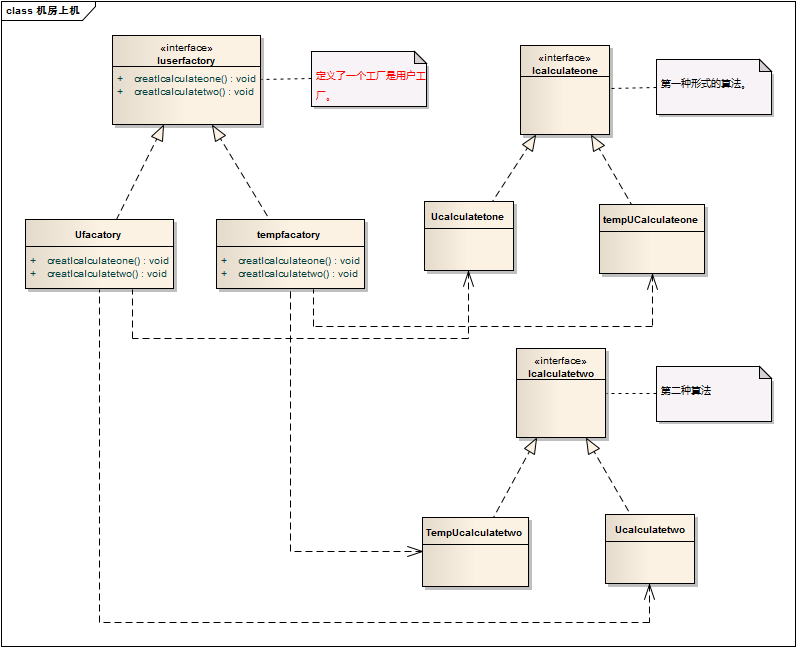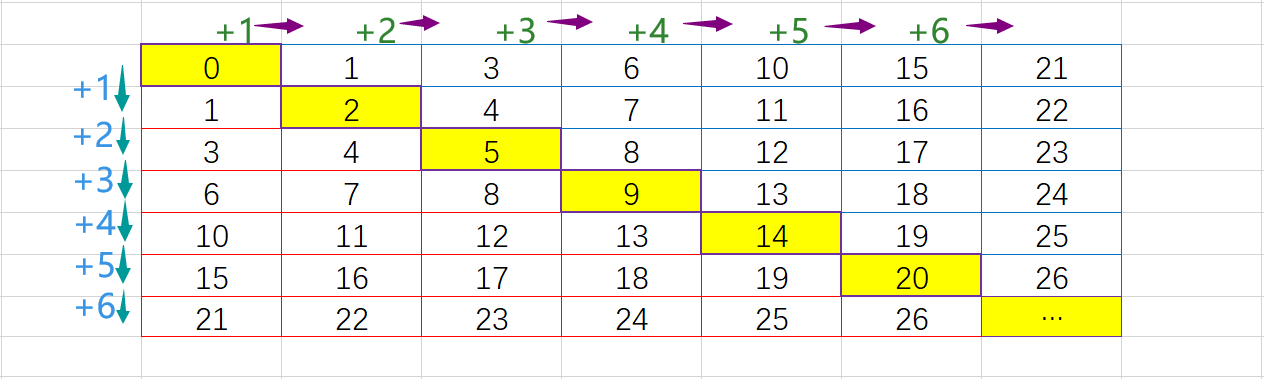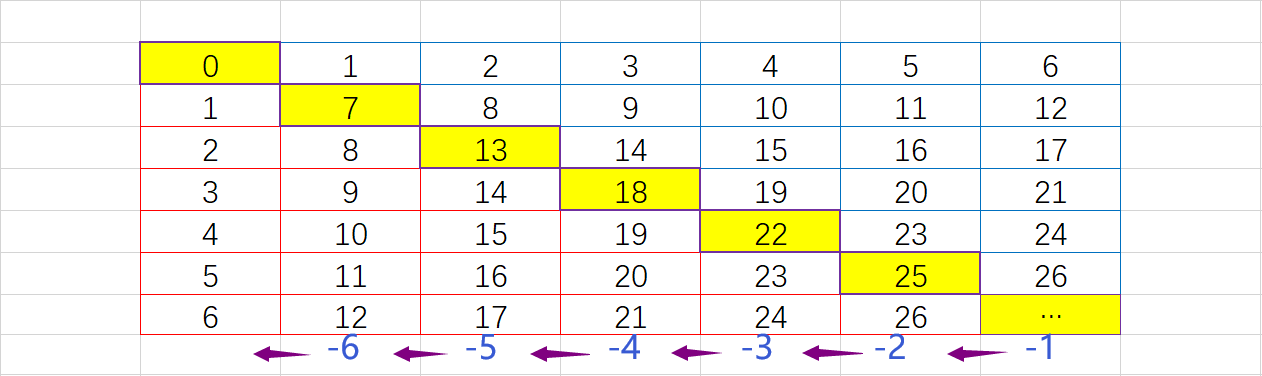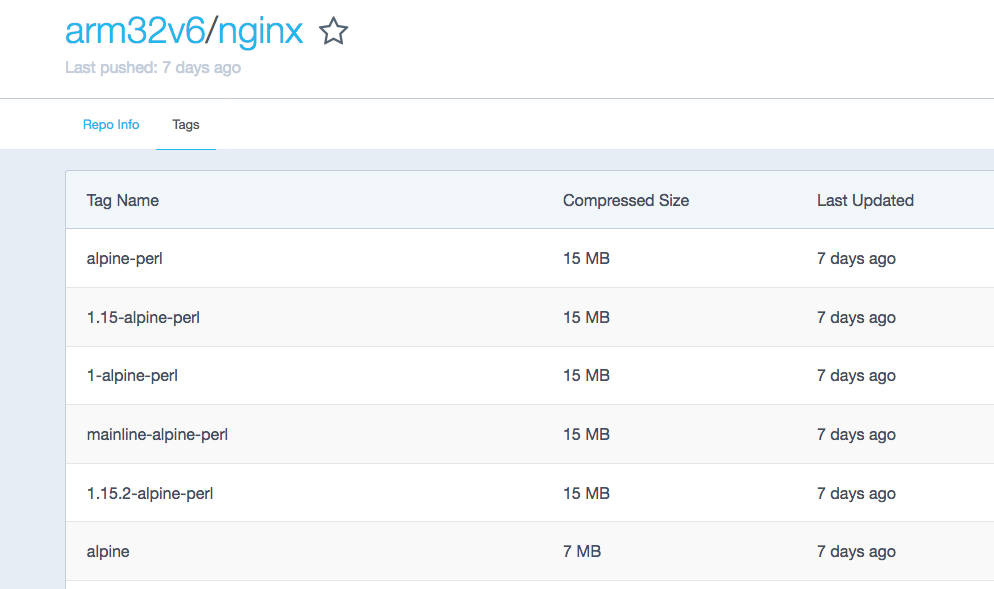• 从策略模式想到抽象工厂的时间不长，但是如何策划类与类之间的关系，之间如何进行传参数，我了很长的时间，看来对于设计模式而，不仅仅是适用的场景，我们也要更加注重设计模式类之间的关系！
前言：
其实在敲机房收费系统的时候无论是手动下机，还是系统在自动下机，还是在选择全部和部分下机的时候都用到了计算上下机的时间算法，其实一提到算法，我们很自然的就想到了策略模式，但是在仔细分析了上下机算法的情况的之后，我觉得在这里用抽象工厂模式更加的适合。
内容：
首先说一下我计算钱的一种方式，我的计算单位时间是30分钟，每隔一个单位时间固定用户就是2元，临时用户就是3元。在上机时间与单位时间之比如果有余数，那么就是上机时间除以单位时间加上1除以单位金额，否则的话就是上机时间除以单位时间乘以单位金额。简单说就是：
用户=固定用户
上机时间mod单位时间>0 那么 （上机时间/单位时间+1）*单位金额
否则（上机时间/单位时间）*单位金额
用户=临时用户
上机时间mod单位时间>0 那么 （上机时间/单位时间+1）*单位金额
否则（上机时间/单位时间）*单位金额
反应到抽象工厂模式的uml图如下：相关的代码如下：

#Region "计算算法-抽象工厂方法。"
'第一种算法，算法：时间/单位时间是整数是整数的，那么就让时间乘以价格

Public Interface Icalculateone
Function calculateone(basicdatalist As List(Of Model.basicdata), consumetime As Integer)

End Interface
Public Class tempUCalculateone : Implements Icalculateone

Public Function calculateone(basicdatalist As List(Of Model.basicdata), consumetime As Integer) As Object Implements Icalculateone.calculateone
Dim temprate As Decimal = basicdatalist(0).TempRate
Dim unittime As Integer = basicdatalist(0).Unittime
Dim product As Decimal = temprate * consumetime / unittime
Return product

End Function
End Class
Public Class Ucalculatetone : Implements Icalculateone

Public Function calculateone(basicdatalist As List(Of Model.basicdata), consumetime As Integer) As Object Implements Icalculateone.calculateone
Dim rate As Decimal = basicdatalist(0).Rate
Dim unittime As Integer = basicdatalist(0).Unittime
Dim product As Decimal = rate * consumetime / unittime
Return product
End Function
End Class
'第二种算法，这种算法是余数部位0，那么让结果加1
Public Interface Icalculatetwo
Function calculatetwo(basicdatalist As List(Of Model.basicdata), consumetime As Integer)
End Interface
'临时用户的第一种算法。
Public Class TempUcalculatetwo : Implements Icalculatetwo

Public Function calculatetwo(basicdatalist As List(Of Model.basicdata), consumetime As Integer) As Object Implements Icalculatetwo.calculatetwo
Dim tempRate As Decimal = basicdatalist(0).TempRate
Dim unittime As Integer = basicdatalist(0).Unittime
Dim product As Decimal = tempRate * (consumetime \ unittime + 1)
Return product
End Function
End Class
'固定用户的第一种算法。
Public Class Ucalculatetwo : Implements Icalculatetwo
Public Function calculatetwo(basicdatalist As List(Of Model.basicdata), consumetime As Integer) As Object Implements Icalculatetwo.calculatetwo
Dim Rate As Decimal = basicdatalist(0).Rate
Dim unittime As Integer = basicdatalist(0).Unittime
Dim product As Decimal = Rate * (consumetime \ unittime + 1)
Return product
End Function
End Class
'用户工厂
Public Interface Iuserfactory
Function creatIcalculateone() As Icalculateone
Function creatIcalculatetwo() As Icalculatetwo
End Interface
'临时用户工厂。
Public Class tempfacatory : Implements Iuserfactory
Public Function creatIcalculateone() As Icalculateone Implements Iuserfactory.creatIcalculateone
Return New tempUCalculateone()
End Function

Public Function creatIcalculatetwo() As Icalculatetwo Implements Iuserfactory.creatIcalculatetwo
Return New TempUcalculatetwo()
End Function
End Class
'固定用户工厂。
Public Class Ufacatory : Implements Iuserfactory
Public Function creatIcalculateone() As Icalculateone Implements Iuserfactory.creatIcalculateone
Return New Ucalculatetone()
End Function

Public Function creatIcalculatetwo() As Icalculatetwo Implements Iuserfactory.creatIcalculatetwo
Return New Ucalculatetwo()
End Function
End Class
#End Region


遇到算法的时候我们理应想到的是策略模式，但是如果在根据具体的情况加以分析，可能会有更适合的模式。还有在外观层的时候我们可以写一个简单的的的switch case 语句进行简单的判断，然后再UI层调用即可，具体的代码就不呈现了，不影响大家的思路！
总结：
从策略模式想到抽象工厂用的时间不长，但是如何策划类与类之间的关系，之间如何进行传参数，我用了很长的时间，看来对于设计模式而言，不仅仅是适用的场景，我们也要更加注重设计模式类之间的关系！


展开全文• 今天写页面时了一个onepage scroll的插件，就是滑一下滚动一页，发现屏幕高度...我使用百分比设的上下间距，就上下间距而，我这种情况是百分比设好呢还是px设好呢？ 问题有点多，希望各位大神伸出援助之手~~~~css 插件
• 对于三角而：索引每层都是顺序递增，且第一层只含有一个元素，第二次含有两个元素，以此类推…故只需确定起点就可由列表转为矩阵。起点的递增便可以理解为二阶导数是1的一个函数。起点函数表示即f(y+1)=f(y)+y...
PS:此处使用的表示方式只是邻接矩阵和只存半三角矩阵这两种。
邻接矩阵的转化方法:

下三角压缩列表转化邻接矩阵(设矩阵的阶数为$N$)对于下三角而言：索引每层都是顺序递增，且第一层只含有一个元素，第二次含有两个元素，以此类推…故只需确定起点就可由列表转为矩阵。起点的递增便可以理解为二阶导数是1的一个函数。起点用函数表示即$f(y+1)=f(y)+y+1,f(0)=0,此处y表示第几行.$
对于上三角而言：由于是对称的关系，起点便是变为由该层的对角元素开始，则起点函数便是$g(x+1)=g(x)+x+2,x∈[0, N-1),g(0)=0，此处x表示第几行$,则元素的递增元素函数为$f(y+1)=f(y)+y+1,y∈(x, N-1),初始状态下f(y)=g(x),此处y表示第几列,x表示第几行$
时间复杂度是$O(n)$
…

下三角起点函数:  $f(y+1)=f(y)+y+1,f(0)=0,y∈[0,N-1),此处y表示第几行$
下三角元素函数:  $g(x+1)=g(x)+1, x∈[0, y-1),g(0)=f(y),此处x表示第几列,y表示第几行$
上三角起点函数:  $g(x+1)=g(x)+x+2,x∈[0, N-1),g(0)=0,此处x表示第几行$
上三角元素函数:  $f(y+1)=f(y)+y+1,y∈(x, N-1),初始状态下f(y)=g(x),此处y表示第几列,x表示第几行$

上三角压缩列表转化邻接矩阵(设矩阵的阶数为$N$)对于上三角而言：每层都是顺序递增的，只需确定矩阵的总阶数，就可上三角元素和下三角元素进行拼接，完成转换。由于半三角列表满足等差数列，故总阶数函数是$N=f(length)=\frac {\sqrt{1+8*length}-1}{2}$。有了总阶数，就可算出每一层对角元素的值，即起点函数为$g(x+1) = g(x) + (N-x),x∈[0, N-1),g(0) = 0, 此处x表示第几行$。
对于下三角而言：起点函数$f(y)=y, y∈[0, N),此处y表示第几行$,而该行的元素递增是一个二阶导数为-1的函数，元素函数为$g(x+1) = g(x) + [N-(x-1)], x∈[0, y-1), 初始状态下g(x)=f(y), 此处x表示第几列,y表示第几行$
时间复杂度是$O(n)$
…

下三角起点函数:  $f(y)=y, y∈[0, N), 此处y表示第几行$
下三角元素函数:  $g(x+1) = g(x) + [N-(x-1)], x∈[0, y-1), 初始状态下g(x)=f(y), 此处x表示第几列,y表示第几行$
上三角起点函数:  $g(x+1) = g(x) + (N-x),x∈[0, N-1),g(0) = 0, 此处x表示第几行$
上三角元素函数:  $f(y+1)=f(y)+y+1,y∈(x, N-1),初始状态下f(y)=g(x),此处y表示第几列,x表示第几行$

无论是上三角还是下三角，只需在每一行以对角元素为准，向右输出$b$个元素，向左输出$a$个元素，只要满足$a+b+1=N$即可转换为邻接矩阵。$设row=行数,矩阵的阶数为N$

$a=[0, row]$
$b=[N-row-1, N)$

简单代码实现:
class SymmetryGraph:
def __init__(self, matrix, shape_type):
"""
初始化图
:param matrix: 邻接矩阵、上三角形式或下三角形式
:param shape_type: matrix、list_up、list_down
"""
# 图
self.data = matrix
# 表示形式
self.type = shape_type
# 节点数
if self.type == 'matrix':
self.n = len(self.data)
else:
spot_num = ((8 * len(self.data) + 1) ** 0.5 - 1) / 2
if spot_num == float(int(spot_num)):
self.n = int(spot_num)
else:
raise TypeError('data is not a symmetry graph')

def info(self):
"""
显示对称矩阵
:return:
"""
print('=' * self.n * 3)
if self.type == 'matrix':
for i in self.data:
print(str(i).replace(',', ' '))
elif self.type == 'list_up':
num = 0
for i in range(self.n):
row = self.data[num: num + self.n - i]
print('[' + '   ' * (self.n - len(row)) + str(row)[1:].replace(',', ' '))
num += self.n - i
elif self.type == 'list_down':
num = 0
for i in range(self.n):
row = self.data[num: num + i + 1]
print(str(row)[:-1].replace(',', ' ') + '   ' * (self.n - len(row)) + ']')
num += i + 1

def to_convert(self, mode='list', spilt='up'):
"""
转化对称矩阵为上三角或下三角数组
======================================================================
matrix = [
[0, 0, 0, 0, 1],
[0, 0, 1, 1, 0],
[0, 1, 0, 1, 0],
[0, 1, 1, 0, 1],
[1, 0, 0, 1, 0]
]
m = SymmetryGraph(matrix)
m.to_convert(mode='list', spilt='up')    # 将对称矩阵转化为上三角数组
m.to_convert(mode='list', spilt='down')  # 将对称矩阵转化为下三角数组

matrix_up = [0, 0, 0, 0, 1, 0, 1, 1, 0, 0, 1, 0, 0, 1, 0]
m = SymmetryGraph(matrix_up)
m.to_convert(mode='matrix', spilt='up')    # 将上三角数组转化为对称矩阵

matrix_down = [0, 0, 0, 0, 1, 0, 0, 1, 1, 0, 1, 0, 0, 1, 0]
m = SymmetryGraph(matrix_down)
m.to_convert(mode='matrix', spilt='down')  # 将下三角数组转化为对称矩阵
======================================================================
:param mode: 转换的目标: list, matrix
:param spilt: 数组的分割: up, down
:return: None
"""
if not self.data:
raise TypeError('graph is empty')
temp = list()
if mode == 'list' and self.type == 'matrix':
if spilt == 'up':
for row in range(len(self.data)):
temp.extend(self.data[row][row:])
self.data = temp
self.type = 'list_up'
elif spilt == 'down':
for row in range(len(self.data)):
temp.extend(self.data[row][:row + 1])
self.data = temp
self.type = 'list_down'
elif mode == 'matrix' and 'list' in self.type:
if spilt == 'up':
i_0, j_0 = 0, 0
for i in range(self.n):
j_0 = i_0
temp.append(self.data[i_0: i_0 + self.n - i])
i_0 += self.n - i
for j in range(len(temp[i]), self.n):
j_0 = j_0 - j
temp[i].insert(0, self.data[j_0])
self.data = temp
elif spilt == 'down':
i_0, j_0 = 0, 0
for i in range(self.n):
row = list()
i_0 += i
for j1 in range(i_0, i_0 + i + 1):
row.append(self.data[j1])
j_0 = j1
for j2 in range(i + 1, self.n):
j_0 += j2
row.append(self.data[j_0])
temp.append(row)
self.data = temp
self.type = 'matrix'
elif mode == 'list' and 'list' in self.type:
self.to_convert(mode='matrix', spilt=self.type.replace('list_', ''))
self.to_convert(mode='list', spilt=spilt)

def conn_status(self, p1, p2):
if self.type == 'matrix':
return self.data[p1][p2]
elif self.type == 'list_up':
[p1, p2] = sorted([p1, p2])
pos = (p1 * (2 * self.n - p1 - 1)) // 2 + p2
return self.data[pos]
elif self.type == 'list_down':
[p1, p2] = sorted([p1, p2], reverse=True)
pos = (p1 * (p1 + 1)) // 2 + p2
return self.data[pos]

matrix0 = [
[0, 0, 0, 0, 1, 1],
[0, 0, 1, 1, 0, 0],
[0, 1, 0, 1, 0, 1],
[0, 1, 1, 0, 1, 1],
[1, 0, 0, 1, 0, 1],
[1, 0, 1, 1, 1, 0]
]
# matrix_down = [0, 0, 0, 0, 1, 0, 0, 1, 1, 0, 1, 0, 0, 1, 0, 1, 0, 1, 1, 1, 0]
# matrix_up = [0, 0, 0, 0, 1, 1, 0, 1, 1, 0, 0, 0, 1, 0, 1, 0, 1, 1, 0, 1, 0]
m = SymmetryGraph(matrix=matrix0, shape_type='matrix')
m.info()
m.to_convert(mode='list', spilt='down')
m.info()
m.to_convert(mode='list', spilt='up')
m.info()
status = m.conn_status(4, 0)
print('点4和点0的连通性是:', status)

运行结果
==================
[0  0  0  0  1  1]
[0  0  1  1  0  0]
[0  1  0  1  0  1]
[0  1  1  0  1  1]
[1  0  0  1  0  1]
[1  0  1  1  1  0]
==================
[0               ]
[0  0            ]
[0  1  0         ]
[0  1  1  0      ]
[1  0  0  1  0   ]
[1  0  1  1  1  0]
==================
[0  0  0  0  1  1]
[   0  1  1  0  0]
[      0  1  0  1]
[         0  1  1]
[            0  1]
[               0]
点4和点0的连通性是: 1



展开全文矩阵
• 题目解析这个题目而之前好像是遇到过，所以很快就过了，题目的意思应该说比较明确的，就是要求从的将节点输出出来，需要注意的地方是，同一层的节点要从左向右边依次的输出。所以这个题目应该说很明显是一个...
题目描述从上往下打印出二叉树的每个节点，同层节点从左至右打印。题目解析这个题目而言之前好像是遇到过，所以很快就过了，题目的意思应该说比较明确的，就是要求从上到下的将节点输出出来，需要注意的地方是，同一层的节点要从左向右边依次的输出。所以这个题目应该说很明显是一个层次遍历的题目。解题思路层次遍历可以用栈来实现，先把数据入栈，然后出栈，出栈的同时，将其左右子节点也入栈，按照这个题目的意思如果用栈来实现应该是右侧的子节点先入栈，然后左侧的子节点入栈，先出左侧子节点，再将该节点的子节点入栈,,,,那么本题中给出的是List的方式，那很好办，而且应该说更好办，让自己来实现这个栈的功能，通过ArrayList数组的方式，从尾部插入相当于入栈了，从0处删除相当于出栈了，也就是靠这个思路，直到整个树中的数据都被存入这个栈中去，并且栈中也已经遍历完了的时候，那么这期间所遍历过的元素的集合就是要求的集合了。代码实现import java.util.ArrayList;
/**
public class TreeNode {
int val = 0;
TreeNode left = null;
TreeNode right = null;

public TreeNode(int val) {
this.val = val;

}

}
*/
public class Solution {
public ArrayList<Integer> PrintFromTopToBottom(TreeNode root) {

ArrayList<Integer> printTree = new ArrayList<Integer>();
ArrayList<TreeNode> temp = new ArrayList<TreeNode>();

if(root == null) {
return printTree;
}

temp.add(root);
while(temp.size() > 0) {

TreeNode node = temp.get(0);
printTree.add(node.val);
temp.remove(0);
if(node.left != null) {
temp.add(node.left);
}
if(node.right != null) {
temp.add(node.right);
}
}
return printTree;

}
}
展开全文• 但当 时 并 没 有 引 起 人 们 的 注 意 ,直 到 1994年 半 年 ,Internet的 迅猛发 展 ,环 球 信 息 网 WWW的 快 速 增 长 ,促 进 了 Java 语 研 制 的 进展 ,使 得 它 逐 渐 成为 Int ernet 受 欢 迎 的 开 ...
• docker对于学习和测试一些常用软件还是非常有帮助的，最近尝试nginx的配置，光看文档还是缺乏上手经验的，恰好手有个树莓派加上自己的电脑，刚好可以来尝试树莓派的代理功能。目前树莓派安装docker已经有了...
docker对于学习和测试一些常用软件还是非常有帮助的，最近尝试下nginx的配置，光看文档还是缺乏上手经验的，恰好手上有个树莓派加上自己的电脑，刚好可以来尝试下树莓派nginx的代理功能。目前树莓派上安装docker已经有了脚本，所以安装只需要

curl -sSL https://get.docker.com | sh

但是对于镜像而言我们是不能直接拉取nginx:latest的，原因在于所有树莓派都是基于armv6架构（可以用uname -a查看）而大部分的主流镜像都是建立在pc的cpu架构上的，所以即便拉取成功运行时还是会直接terminate退出的。当然镜像也不能随便从非官方途径获取，因为这样很容易让你的设备成为DDOS攻击的傀儡。官方的github，对于树莓派而言我们也能从中找到对应的仓库，可以从该仓库中找到arm32v6/nginx，这就是能在树莓派上运行的镜像，所以我们可以先获取镜像

docker pull arm32v6/nginx

我第一次拉取该镜像的时候直接报错了

Using default tag: latest
Error response from daemon: manifest for arm32v6/nginx:latest not found

明明可以从仓库中看到这个镜像docker pull却提示不存在。后来我人为可能跟镜像的tag有关，默认的是latest也就是最后一个版本。但现在提示不存在也就是说我们得指定tag了在tags栏中我选择了alpine-perl

docker pull arm32v6/nginx:alpine-perl

指定tag后果然就成功了。接下来要运行镜像（同样需要指定tag）

docker run -p 80:80 --name nginx --rm arm32v6/nginx:alpine-perl

我们并没有用-d让其后台运行，所以终端会继续存在并暂时没有任何输出。打开浏览器地址栏中输入运行镜像的机器ip，能看到Welcome to nginx!基本上就没有问题了，并且刚刚的终端也会有输出信息，现在可以直接CTR-c关掉这个容器了（用了–rm启动的容器在退出时相应的容器也会被删除）。目前来说仅仅是在树莓派上运行了nginx，我们可以尝试修改nginx的配置文件来达到不同的目的，这里做个小实验

*
linux电脑
树莓派

ip地址
192.168.1.8
192.168.1.9

新建文件
~/nginx_test/test1
~/nginx_test/test2

分别在两个nginx_test文件夹中运行python -m SimpleHTTPServer来建立两个简单的http服务器。运行成功后浏览器地址栏输入192.168.1.8:8000可以看到test1文件，192.168.1.9:8000可以看到test2文件。现在在树莓派上启动nginx（-d让其后台运行）

docker run -p 80:80 -d --name nginx --rm arm32v6/nginx:alpine-perl

用docker ps -a 得到容器ID并登陆容器

pi@raspberrypi:~ \$ docker exec -it 容器ID /bin/sh
/ # 

在nginx的配置文件/etc/nginx.conf中’ include /etc/nginx/conf.d/*.conf; ‘的上一行加入规则(include 这一行是通配原则可以注释掉, 这里我们采取注释)

    upstream backend {
server 192.168.1.8:8000;
server 192.168.1.9:8000;
}

server {
listen 80;
location = / {
proxy_pass http://backend;
}

location = /test1 {
rewrite . http://192.168.1.8:8000;
}

location = /test2 {
rewrite . http://192.168.1.9:8000;
}

}

#include /etc/nginx/conf.d/*.conf; 

保存配置文件后需要执行

nginx -s reload

使得配置文件生效，（容器中直接修改文件，当容器删除后重新从镜像运行会丢失这些修改的内容，可以使用docker commit [OPTIONS] CONTAINER [REPOSITORY[:TAG]]  提交一个新的image来保存这些修改）
浏览器地址访问192.168.1.9并刷新就可以看到test1和test2交替出现了，如果访问192.168.1.9/test1则锁定访问在test1，192.168.1.9/test2则锁定访问在test2。当然这里只是做个小测验，所以规则比较简单，仅仅做个入门而已^^
展开全文• 向上取整,运算称为 Ceiling，数学符号⌈⌉ （有起止，开口向）表示,。 向取整,运算称为 Floor，数学符号⌊⌋（有起止，开口向上）表示。 注意，向上取整和向取整是针对有浮点数而的; 若整数向上...
• 本文介绍了一种 C 语言实现 P C 机与单片机... 采 串 行 异 步 方 式 及 和 校验发送和接收数据， 位机采用 C 语 编 程 。 经 试 实 验 证 明 方 法 适 于 多 种不 同 的 串 行 通 信 控 制 系 统 。
• 作为前端或网页开发者而，在IE6做CSS调试在之前简直是一个噩梦。作为前端或网页开发者而，但这又是无法回避的事情。某日从红茶那边听说了Firebug Lite这个好东西，可以在不装插件的情况，自由的对IE各个版本...
• 在我前面的文章里有一篇如何去编译arm版golang, 但是就这个项目而, 我忽略了一个问题: golang调用c代码的时候, 需要指定gcc, 而我所指定的gcc是amd64架构, 就算直接copy到arm板子也不能, 还需要编译arm版gcc,...arm golang linux c语言
• CJ今天太上午太无聊，就了个数独玩，结果没耐心，突发奇想不如写个程序解数独，一次努力，永久福利。于是就着手了。 CJ比较直，就像先写个界面出来看看，结果就是这样。 别说话，吻我。我知道这界面很丑。...java 算法 界面
• 在我看来还是比较多的，现在智能手机的功能很多，对大部分人而能真正用到的功能其实非常有限，很多功能其实用户群体非常小，那么，智能手机到底有哪些功能最没有呢？下面就来具体了解。 手机自带浏览器 现在...
• Mac OS X 系统从 ISO 文件的创建可引导的 USB 启动盘 从U盘启动现在越来越...事实, 它甚至可以比刻录 CD 或 DVD 更便宜, 一旦版本过时就扔掉。 对于大多数 Linux 发行版而, 用于刻录 CD/DVD 的 ISO 可在 in...
• 距离一次发表到现在已经有很长是时间了，趁着国庆放假前的空余时间将半部分完成。客户的实现相比服务端稍难一点，我们需要对图像进行截取。我在实现过程中出现了一些问题，先列举出来，第一程序比较吃内存、第二...C# Winform
• 就web service而，REST要比SOAP（SOAP是标准，不是风格）轻量得多，容易得多。最初开始接触web service的时候，所有的材料上来就是一大堆的名词，SOAP, WSDL,看得头都要大了，后来提出来的REST就容易理解得多，...
• 了windows的150%縮放)</li><li>在解像度偏低的平板看不見poi的界面 見上面那截圖</li><li>Contributor第一位的頭像裂了 http://i.imgur.com/PqW7HVe.png</li><li>平板在旋轉後界面不能自適應而出現白色 ...
• 一、缺少java源文件 如图: 现在想要在main下面增加java源文件，效果图如下所示: ...首先，在main目录新建java文件夹，就是源文件目录， ...然后，右键，选择Mark Directory ...对于IDEA而，你只需要先创建一...
• 于我而   今天PL/SQL Developer启动本地库时，报错ORA-12514:TNS:监听程序当前无法识别连接描述符中请求的服务。。想着自己之前也遇过类似问题，并且在本分类里面有过解决方案。但是尝试sql plus连接本地库...
• 本文是我的同学杨钊（西安电子科技大学软件学院）写的操作系统课程的论文，据他介绍，目前在网上几乎没有介绍即插即的原理方面的资料。...对用户而，只要将外围设备“插”就能使用，不需要具备任何windows microsoft 配置管理 object
• 可能在几年之前，很多...对于开发者而，是时候让你的网站用上Html5了，甚至于移动端的应用都可以基于HTML5移动技术来开发了，下面的一些内容很好的证明了这一点。 　 　首先在Web端，截至2012年4月，IE9在Window浏览器
• 随手点开看了，内容几乎也都大同小异，无非就是几个关键词配几个故事，然后再贩卖焦虑，得出结论：这届年轻人是将被“毁掉”的一代。 相似的文章太多，一不合就被“毁掉”，我们这届年轻人可真是混得太惨了...
• 最近了一段时间的CSS，自我感觉已经可以进行一些初步的CSS布局了，不过对于CSS这个东西而，个人觉得也确实不难，其实只要一样一样搞懂就可以了。 之前学了一段时间的CSS，以为动画在CSS中应该会比较难，不过经过...css 动画
• 对于深度学习而，在数据样例的 batch 运行模型十分常见，这能通过并行计算加速训练，并在每一步有更加平滑的梯度。现在我们就开始，下文会解释篇描述的如何对 stack-manipulation 进行 batch。PyTorch text ...
• 因为对于root用户而，PATH值和普通用户不同，它在普通用户PATH值的基础一般还包含了/sbin和/usr/sbin。所以如果普通用户在执行命令时，如果没有指定命令的完整路经，就不可能执行/sbin目录中的命令。同样，普通...虚拟机 path linux disk
• 　不知道现在润乾报表的同学还多不多，尤其是V4.5，基本不更新了，实际也不提供技术支持了。 　在此抽空分享平时工作中遇到的问题和一些技巧。 　博客新人，写的不好，诸多包含和交流。 转载于:...
• 正解：百度有上下界限制的网络流，发现这是一类问题，以”无源点汇点“这种最为基础和简单，推荐博文，简单的说就是利用对于每个点而 入边下界+入边自由流==出边下界+出边自由流 ，既然给出下界，那么我这个数给......